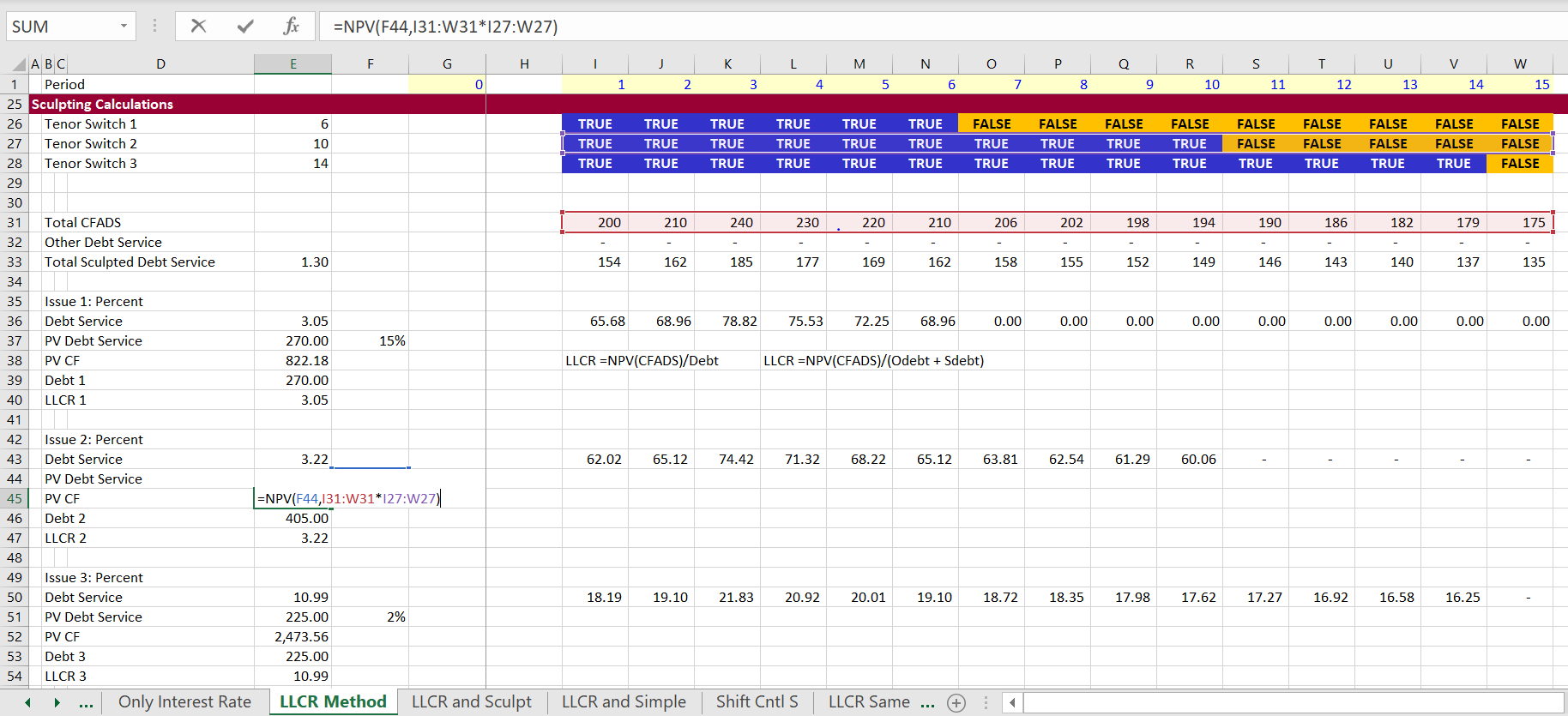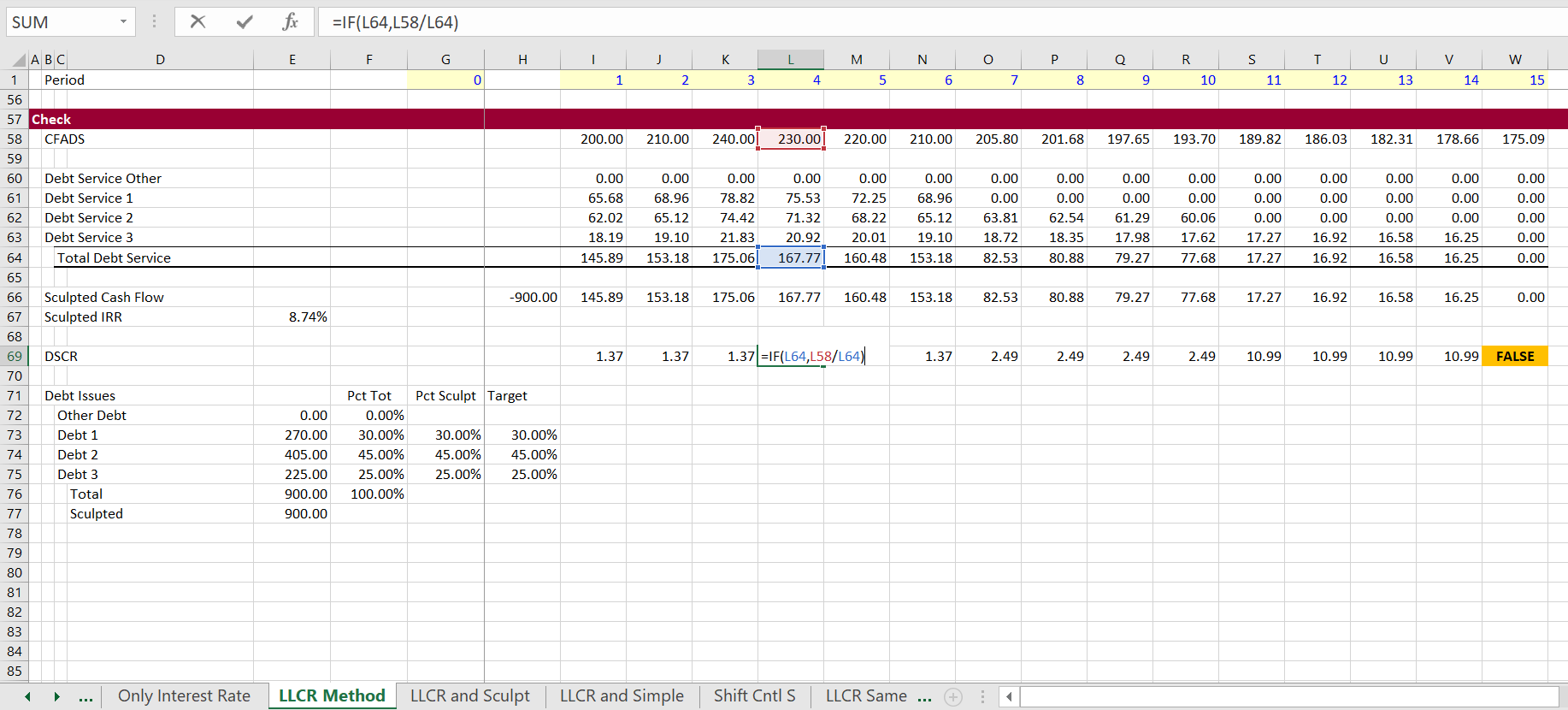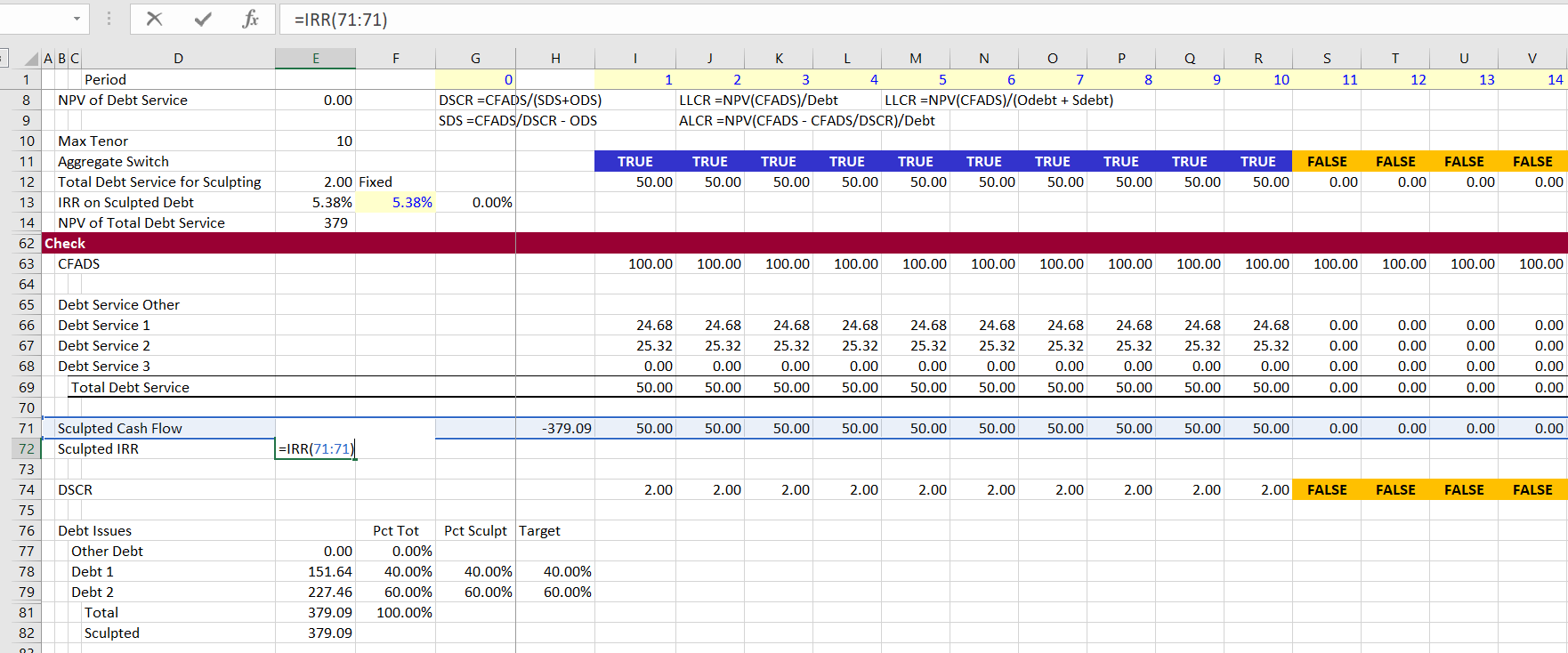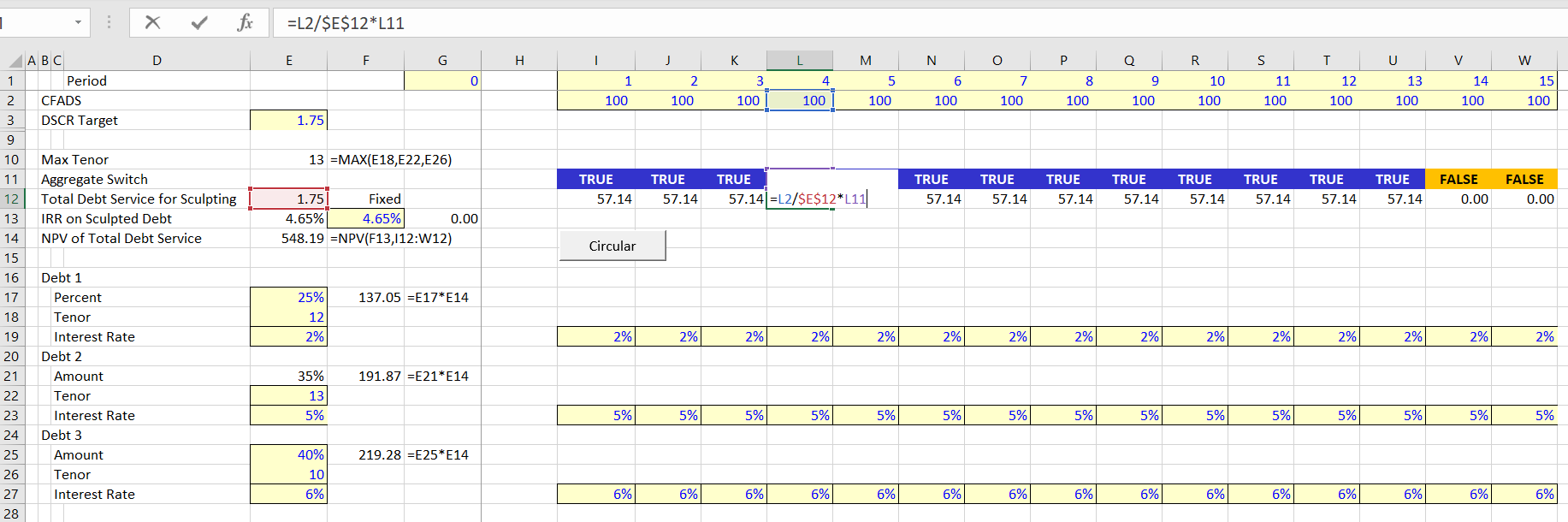# Multiple Sculpted Issues

This page describes debt sculpting in project fiance when there are multiple debt issues all of which are sculpted. In this case allocation of sculpted debt repayment to different issues with different interest rates and different debt tenors, the calculations are more complex. This type of situation with multiple debt issues that are sculpted can occur when there is an issue from a development finance institution as well as an issue from a private bank.  The commercial bank may often be sculpted in the same manner as the development finance institution, but have a short tenure.

## Modelling Multiple Debt Issues All with Sculpted Repayment with Different Tenures and Different Interest Rates Using Capture and Non-Capture Issues

This section moves from fairly basic issues to a difficult issue where you are not given repayment on the non-sculpted debt issues, but you have to compute sculpted repayment on many debt issues.  The mechanics of computing multiple sculpting is more difficult than where you have one sculpted debt issue and one non-sculpted issue as in the case of the balloon payment.  As shown above, the modelling of sculpting is not all that difficult where only one of the debt issues is sculpted.  You can use the formula to derive the sculpting by using the following formula:

Sculpted Issue DS = CFADS/DSCR – Other DS

If other debt issues are sculpted, this formula does not work in a simple manner.  In this section I explain how you can compute sculpted debt repayments and debt service when you have multiple debt issues with different terms (tenures and interest rates). I have put together a file that includes issues of calculating debt service for multiple debt issues in the file below.

With multiple debt issues, I define one debt issue — the issue with the longest tenure — as the capture debt issue.  Other debt issues are defined as the non-capture debt issues. The rule to computed sculpted debt repayment where you have multiple debt issues is the following:

1. Compute the overall debt IRR using all of the sculpted debt issues when you compute the NPV of the CFADS.
2. Compute the LLCR for the non-sculpted debt issues using the interest rate of the debt issue, the tenure of the debt issue and the CFADS to calculate the numerator.  The denominator of the LLCR is the amount of debt which is the NPV of the total debt issue multiplied by the target debt percent.
3. Compute the debt service of the captured debt issue using the target DSCR for overall cash flow and the debt service of all of the other non-captured debt issues.  This applies the formula DS = CFADS/DSCR – Non-capture Debt Service.
4. Compute the IRR using the net debt cash flow that includes the total amount of the debt and the total amount of the debt service.

### 1. Simple Allocation of Cash Flow Method to Compute Sculpted Balances

To introduce the issue I have created a simple example with three debt issues.  Each issue has a different debt tenure and a different interest rate.  Each issue also has a target amount as percent of the total debt.  This set-up of the sculpted debt inputs is shown in the screenshot below.  If there is a loan from a development financial institution, this type of situation with multiple debt issues that are sculpted can occur. If there is also a non DFI, the non DFI may have a shorter tenure and a higher interest rate.  Note that the last debt issue in the screenshot has a tenure of 14 years while the first issue has a tenure of 8 years.In the above screenshot I entered the target sculpting for each debt issue.  This had to be computed twice to recognise that after the first debt is finished, the percentages will change.  Ultimately, when there is only one debt issue outstanding, all of the cash flow is allocated to that issue.  These percentages are applied to the CFADS and then the sculpting is made with the normal NPV formula. The calculation of sculpting from the allocated CFADS to each issue is shown in the screenshot below.  The NPV of the debt service gives you the total amount of debt as usual. If the interest rate is lower the NPV of the debt is higher. If the tenure is longer the NPV is higher.  All of this means that the target percentages do not equal the final debt balance percentages.  This is demonstrated at the bottom of the page.  On the table at the bottom of the page, the target percentages computed from the NPV of debt service are very different from the target percentages.### 2. LLCR Method to Allocate Sculpting for Multiple Debt Issues

I have described how you can compute sculpting if the debt balance is given.  In this case you can use the formula to find the target DSCR to sculpt from a simulated LLCR.  The formula is:

In the example below, the total debt is given as a fixed amount of 900 and it is allocated between three debt issues.  For each issue, the PV of cash flow over the tenure of the debt is computed.  Then, the total debt of 900 is allocated among the three issues. This defines the target amount of debt.  The LLCR is computed for each issue from the PV of Cash Flow as well as the target amount of debt.  This computation of the LLCR is illustrated in the screenshot below.  The formula in the screenshot illustrates how you can put a formula (with TRUE/FALSE multiplied by the CFADS) into the NPV formula.  Note that the LLCR is very high because the total cash flow is compared each separate debt issue.Results of the LLCR method are shown in the screenshot below. In this screenshot the good news is that the amount of debt is the target debt.  But don’t get too happy.  The very bad news is that the DSCR does not match either the LLCR on the overall debt or the target DSCR.  Note that the DSCR begins at a level of 1.37. But after the first debt issue is finished, the DSCR increases to 2.49.  Then, when only the last issue is outstanding, the DSCR is 10.99. If you allocate debt in this way the fact that the DSCR is not constant demonstrates that the method does not work.### 3. LLCR on One Issue and Remainder Formula for Second Issue

The next method is getting a bit closer, but it still does not quite work.  In a case with different interest rates and only two debt issues, you can reach both the target DSCR and the target percentages of debt. The method starts by establishing the overall debt size from the target DSCR.  The debt size is derived by computing the NPV just like in any of the debt sizing cases. This interest rate is used in the formula Debt = NPV(Debt IRR, Debt Service) where Debt Service = CFADS/Target DSCR. But to compute the debt size, you need a discount rate. The discount rate is the overall IRR on debt is computed from evaluating the total amount of debt versus the debt service from all of the debt issues.

The method of establishing the LLCR fror one issue and using the debt service formula for the second issue works when the tenure is the same for both issues because of a circular reference problem. The screenshot below illustrates the approach.  The first debt issue is computed with the LLCR using the method discussed in the prior section.  The PV of cash flow is computed and then the LLCR is derived from the target amount of the debt: LLCR = PV Cash/Debt. Using this LLCR to compute debt service produces debt service for the first debt issue and it also yields the target debt size for the first issue.  For the second issue, the formula for debt service of is DS = CFADS/DSCR – Other DS, where the Other DS includes the debt service from the first debt issue.  I label this debt issue as the capturing debt issue. When you compute the NPV of this cash flow for the second issue, you get the same amount as the target debt percent multiplied by the total debt.

A complexity with this method is that works from the IRR on the overall debt. This is needed because the IRR on the debt that is used for sculpting.  But the IRR on debt cannot be computing until the debt issues are evaluated.  This causes a circular reference which will be discussed in detail below.  This is shown in the second screenshot below.The next screenshot demonstrates the results of this combination of LLCR and formula method.  In this method, the target DSCR of 2.0 is achieved and the target debt amounts for the two issues are also achieved as shown on the bottom of the screenshot.  To make this computation work, the debt IRR is computed from the debt service of the two debt issues.  This IRR is 5.38%.  But the IRR is not known until the debt service of the two issues is computed. The IRR is copied and pasted into the calculation for the NPV of overall debt service.### 4. LLCR on and Maximum Tenure with Circular Resolution

The multiple sculpting issue can be solved when you set-up the analysis to first establish which issue has the longest tenure.  This debt issue with the longest debt tenure is labeled the capturing debt issue as in the last section. Once the maximum debt tenure is established, the process of computing sculpting with multiple debt issues involves the following steps:

Step 1: Compute the maximum debt tenure from the debt tenure of all of the issues

Step 2: Insert a discount rate and compute the total debt from the present value of the debt service derived from the target DSCR.  The discount rate is the debt IRR

Step 3: Compute that size of each debt issue from the given percent of debt.  This is shown in the screenshot below.Step 4: For the non-capture debt issues, compute the present value of cash flow at the interest rate of the debt issue and account for the tenure of the debt.  This is shown in the screenshot below.

Step 5: For the non capturing debt issues, compute the LLCR in order to derive the debt service for the different debt issues.  The LLCR is computed from target amount of the debt and the present value of the cash flow.Step 6: Compute the debt service for the non-capture debt issues using the LLCR and the debt tenure.

Step 7:  For the capture debt issue, the debt service is computed as DS = CFADS/Target DSCR – Other Debt Service; where the other debt service in the formula includes debt service from the sculpted issues that are not the capturing debt issue.  This is illustrated in the screenshot above.

Results of a case with changing interest rates and also a debt issue with non-sculpted debt is shown in the screenshot below.  For this case, the non-sculpted debt service is included in the subtraction from the capture debt issue.  To implement the changing interest rate, use the SUMPRODUCT function with and interest rate index.